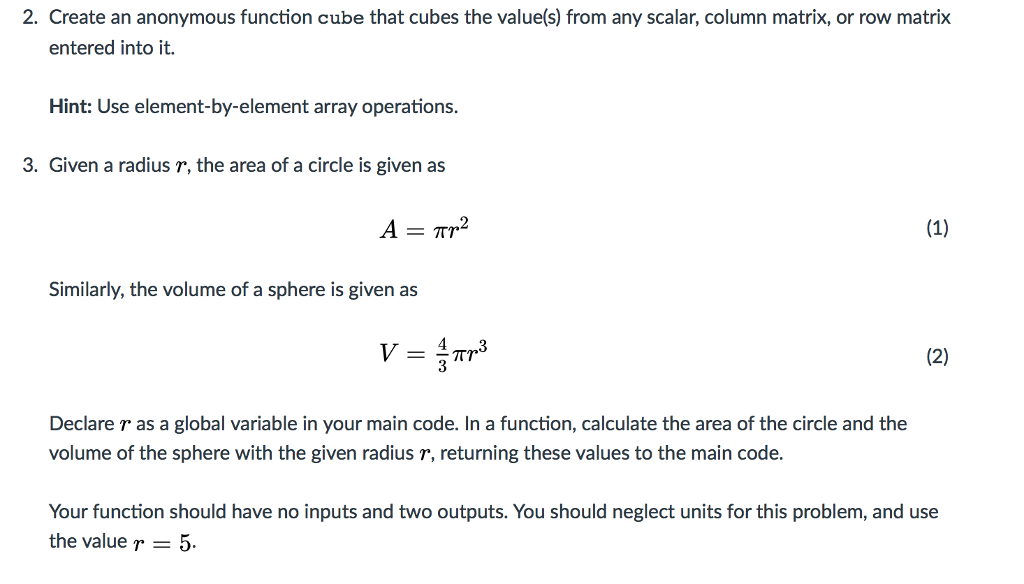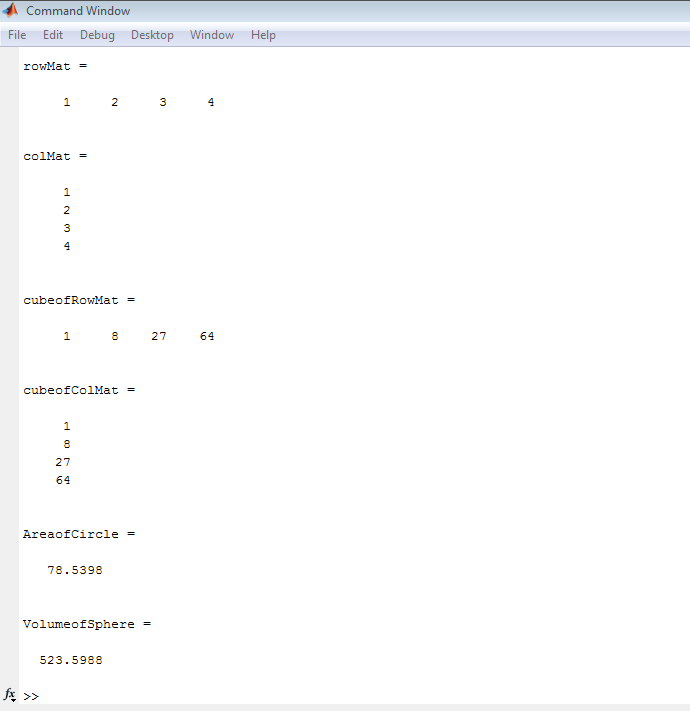# Answered! Create an anonymous function cube that cubes the value(s) from any scalar, column matrix, or row matrix entered…

This is the third time I post this question !!! PLEASE RUN THE CODE BEFORE YOU SUBMIT THE SOLUTION. ( MATLAB )Don't use plagiarized sources. Get Your Custom Essay on
Answered! Create an anonymous function cube that cubes the value(s) from any scalar, column matrix, or row matrix entered…
GET AN ESSAY WRITTEN FOR YOU FROM AS LOW AS \$13/PAGE

Create an anonymous function cube that cubes the value(s) from any scalar, column matrix, or row matrix entered into it. Hint: Use element-by-element array operations. 3. Given a radius r, the area of a circle is given as A Tr2 (1) Similarly, the volume of a sphere is given as (2) Tr Declare r as a global variable in your main code. In a function, calculate the area of the circle and the volume of the sphere with the given radius r, returning these values to the main code. Your function should have no inputs and two outputs. You should neglect units for this problem, and use the value r 5.

NOTE: A suggested I have used one sript File(mainScript.m) and two function file(areaCircle.m) and

(volumeSphere.m). What you have to do is ceate 3 files and apprpriate codes (given below ) to respective files.

THIS CODE IS PERFECTLY RUNNING. OUTPUT IS ALSO GIVEN. PLease put propercode in proper file

ANY OTHER SUGGESTION IS WELCOME

——————————————————————————————————————————-

% Main script file mainscript.m

%COPY FOLLOWING Coodes (upto END OF MAINSCRIPT) into mainscript.m file

% ———————————————————–
clc;
global r
r=5;
cube=@(x) x.^3;
%% row matrix
rowMat=[1,2,3,4]
% Column matrix
colMat=[1;2;3;4]
% Cube of row matrix
cubeofRowMat=cube(rowMat)
%Cube of row matrix
cubeofColMat=cube(colMat)
%% Call to compute area of circle
AreaofCircle=areaCircle()
%% Call to compute volume of shpere
VolumeofSphere=volumeSphere()

%—– END OF MAINSCRIPT———————————————–

% Create a function file areaCircle.m and Copy Following codes (UPTO END OF area_cilcle ) into this file

% Function File name : areaCircle.m

%————————————————————–

function A = areaCircle()
%areaCircle return the area
% of a circle with given global radius r
global r;
A=pi*r*r;
end

%——————– END OF areaCircle ————————————–

% Create a function file volumeSphere.m and Copy Following codes (UPTO END OF volumeSphere ) into this file

% Function File name : volumeSphere.m

%————————————————————–

function V =volumeSphere()
%volumeSphere return the volume
% of a sphere with given global radius r
global r;
V=pi*r*r*r*4/3;

end

% ———–END OF volumeSphere ———————————————-

%output below